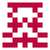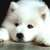2018.1 月赛题解

zerol edited 1 年，5 月前

By ultmaster.

By ultmaster.

By zerol.

D. 华生的围栏

By ultmaster.

• 小于 $3$ 是无解的。
• 对于偶数的情况，只要构造一个长为 $\frac{C}{2}-1$，宽为 $1$ 的长方形即可。
• 奇数是无解的，证明如下：如果存在一个周长为奇数的多边形，我们可以将每一条不跟坐标轴平行的边 $(x_1,y_1)-(x_2,y_2)$ ($x_1 \ne x_2, y_1 \ne y_2$) 替换成对应的折线 $(x_1,y_1)-(x_1,y_2)-(x_2,y_2)$，可以保证做这样的替换后原图周长的奇偶性不变（因为 $a^2+b^2=c^2$ 必然有 $a+b \equiv c \pmod 2$）。现在多边形的所有边都与坐标轴平行，且是一个封闭图形。所以考虑从一个顶点出发，绕个多边形走一圈，每一步向上都有向下的对应，每一步向左都有向右的对应。这样多边形的周长一定是偶数。矛盾。

E 没用的函数

By zerol.

• 如果固定右端点，扩展左端点，那么 gcd 的值最多变化 $logC$ 次（C 为数列中最大的可能值，因为 gcd 每次至少减少一半），而且从左到右 gcd 值是单调递增的。
• 如果右端新加入一个点，那个将原来的每一段的 gcd 都对于新的数取 gcd，再进行去重就能得到新的 gcd 的分段信息。
• 对于每一段，需要求区间最大值，用ST表或者线段树预处理一下即可。
• 复杂度 $O(nlogn)$

F 最小 OR 路径

By zerol.

Easy

• dp[u][k] 表示到结点 u 的代价为 k 的方案是否存在，然后在图上转移（dfs 一下就好了）。
• 可以枚举所有可能的答案，判断连通性。但是如果你想到了这一点，那么 HARD 一定也不在话下。

Hard

• 假设答案的二进制位全是 1，然后从高位到低位考虑，如果将该位置为 0 不破坏连通性的话就置为 0，这样肯定最优。
• 判断连通性可以用 并查集 或者 搜索，反正 $O(M)$ 就行。
• 复杂度 $O(63M)$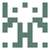之前打错了

2的24次方：16777216

3的23次方：94143178827（逃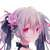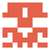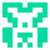A -> C
B -> C
C -> D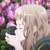$n$ 的确有点小。超时成功是因为 spj 判断时间的常数有点大。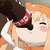C可以到Fib(49)吧